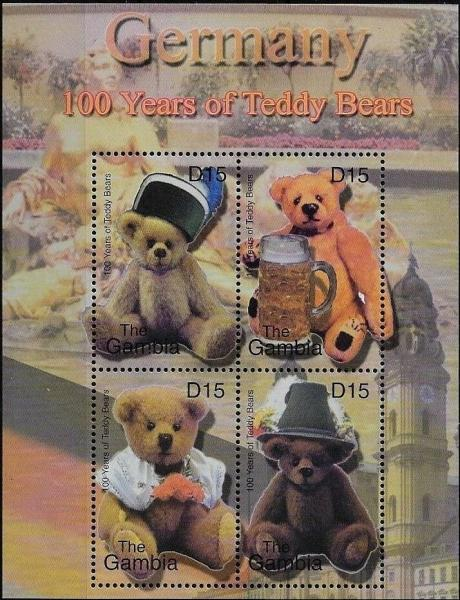## Image Colnect-5757-551-Teddy-Bear-Cent.jpgFull resolution (714 x 931 pixels) Type: sheet Issuer: Gambia Date: 2002 Visibility: public Image owner: none 89 matches: empty sheet (#13979) empty sheet (#13980) empty sheet (#13981) empty sheet (#13982) empty sheet (#13983) empty sheet (#13984) empty sheet (#13985) empty sheet (#13986) empty sheet (#13987) empty sheet (#13988) empty sheet (#13989) empty sheet (#13990) empty sheet (#13991) empty sheet (#13992) empty sheet (#13993) empty sheet (#13994) empty sheet (#13995) empty sheet (#13996) empty sheet (#13997) empty sheet (#13998) empty sheet (#13999) empty sheet (#14000) empty sheet (#14001) empty sheet (#14002) empty sheet (#14003) empty sheet (#14004) empty sheet (#14005) empty sheet (#14006) Moths of the World, sheet of 1 type empty sheet (#53420) empty sheet (#53421) empty sheet (#53422) empty sheet (#53423) empty sheet (#53424) empty sheet (#53425) empty sheet (#53426) empty sheet (#53427) empty sheet (#53428) empty sheet (#53429) empty sheet (#53430) empty sheet (#53431) empty sheet (#53432) empty sheet (#53433) empty sheet (#53434) empty sheet (#53435) empty sheet (#53436) empty sheet (#53437) empty sheet (#53438) empty sheet (#53439) empty sheet (#53440) empty sheet (#53441) empty sheet (#53442) empty sheet (#53443) empty sheet (#53444) empty sheet (#53445) empty sheet (#53446) empty sheet (#53447) empty sheet (#53448) empty sheet (#53449) empty sheet (#53450) empty sheet (#53451) empty sheet (#53452) empty sheet (#53453) empty sheet (#53454) empty sheet (#53455) empty sheet (#53456) empty sheet (#53457) empty sheet (#53458) empty sheet (#53459) empty sheet (#53460) empty sheet (#53461) empty sheet (#53462) empty sheet (#53463) empty sheet (#53464) empty sheet (#53465) empty sheet (#53466) empty sheet (#53467) empty sheet (#53468) empty sheet (#53469) empty sheet (#53470) empty sheet (#53471) empty sheet (#53472) empty sheet (#53473) empty sheet (#53474) empty sheet (#53475) empty sheet (#53476) empty sheet (#53477) empty sheet (#53478) empty sheet (#53479) Crops No visible images cropped from this one. Measurements Resolution is unknown. Source Source name: Colnect File URL: http://i.colnect.net/b/5757/551/Teddy-Bear-Cent.jpg Uploaded December 2, 2019, 8:37 pm by Stan Shebs